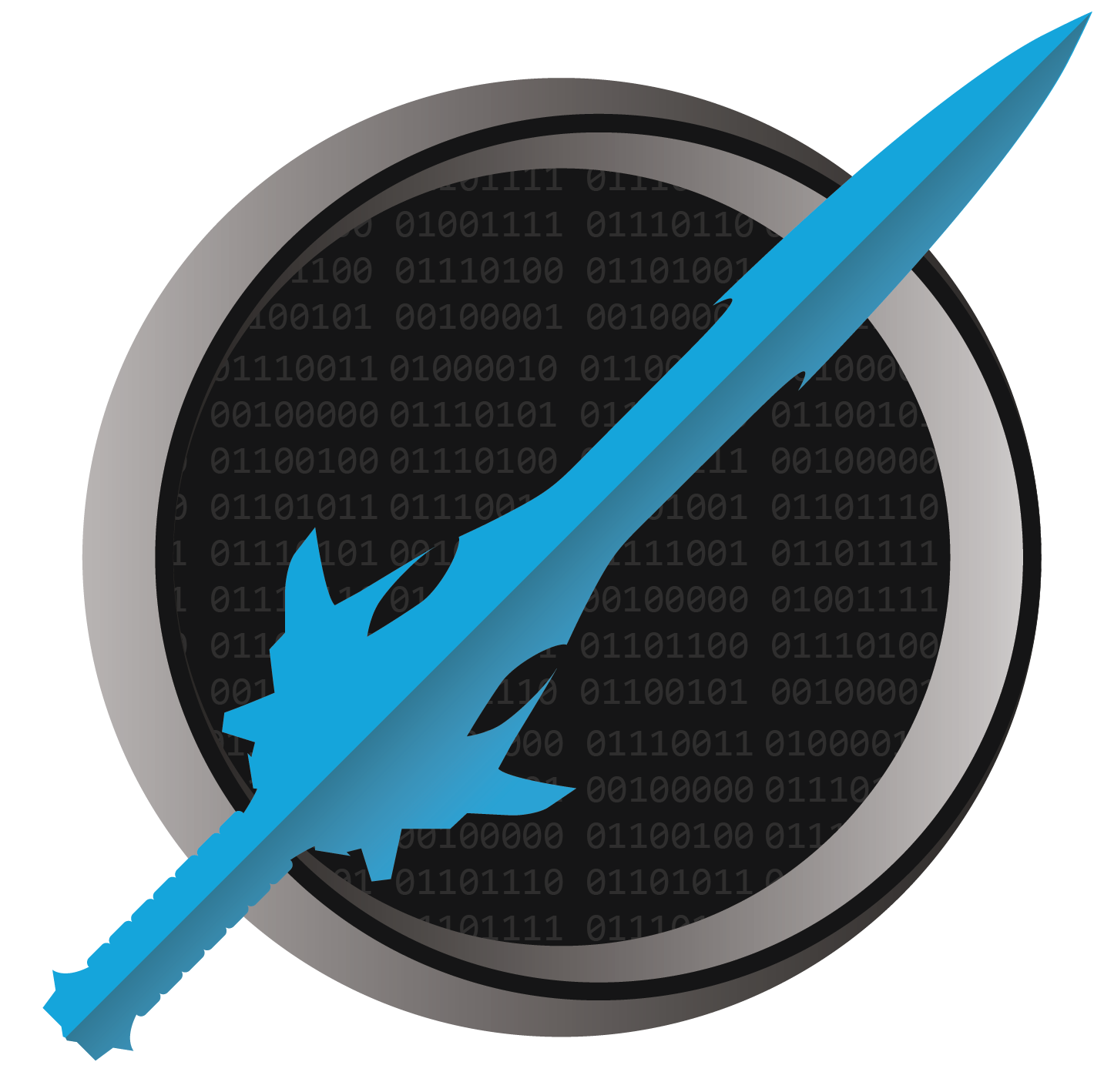It's dangerous to code alone! Take this.

# Laws of Freach

The following is one possible solution to this challenge.

``````using System;

int[] array = new int[] { 4, 51, -7, 13, -99, 15, -8, 45, 90 };

int currentMinimum = int.MaxValue; // Start higher than anything in the array.

foreach(int value in array)
{
if (value < currentMinimum)
currentMinimum = value;
}

Console.WriteLine(currentMinimum);

int total = 0;

foreach(int value in array)
total += value;

float average = (float)total / array.Length;

Console.WriteLine(average);
``````